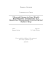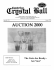# Teknologi Solid State - Universitas Brawijaya

## Transcription

Teknologi Solid State - Universitas Brawijaya
```Teknologi Solid State
mikroelektronika
Eka Maulana, ST, MT, MEng.
Teknik Elektro – Universitas Brawijaya
Pokok Bahasan [Teknologi Solid State]
• Teori, Model dan Struktur Atom
• Sifat dan Klasifikasi Material Solid
• Struktur Kristal (Lattice Bravais)
• Diagram Tingkat Energi (Pita Energi)
• Semikonduktor Intrinsik dan Dopping
• Rapat Muatan dan Mobilitas Muatan
• Mekanisme Transport
• Aplikasi
Teori Atom
• Teori Atom John Dalton
• Teori Atom J. J. Thomson
• Teori Atom Rutherford
• Teori Atom Bohr
• Teori Atom Modern
(Schrodinger)
4
CLASSIFICATION OF SOLIDS
SOLID
MATERIALS
CRYSTALLINE
POLYCRYSTALLINE
AMORPHOUS
(Noncrystalline)
Single Crystal
5
Fisika Solid State
• Menjelaskan sifat atom dan interaksi antara
elektron dengan gaya elektrostatis.
• Formulasi hukum dasar pada material solid.
Crystalline Solid
• Crystalline materials are solids with an atomic structure
based on a regular repeated pattern.
• The majority of all solids are crystalline.
• More progress has been made in understanding the
behavior of crystalline solids than that of non-crystalline
materials since the calculation are easier in crystalline
materials.
• Understanding the electrical properties of solids is right at
the heart of modern society and technology.
Electrical resistivity of three solid
Carbon states
• How can this be? After all, they each contain a system of atoms and
especially electrons of similar density. And the plot thickens:
graphite is a metal, diamond is an insulator and buckminsterfullerene is a superconductor.
They are all just carbon!
CRYSTAL STRUCTURES
 Elementary Crystallography
 Solid materials (crystalline, polycrystalline,





amorphous)
Crystallography
Crystal Lattice
Crystal Structure
Types of Lattices
Unit Cell
 Typical Crystal Structures
(3D– 14 Bravais Lattices and the Seven Crystal System)
SINGLE CRYSTALS


Single crystals have a periodic atomic structure across its
whole volume.
At long range length scales, each atom is related to every
other equivalent atom in the structure by translational or
rotational symmetry
Single Pyrite
Crystal
Amorphous
Solid
Single Crystals
10
POLYCRYSTALLINE SOLIDS





Polycrystalline materials are made up of an aggregate of many small single
crystals (also called crystallites or grains).
Polycrystalline materials have a high degree of order over many atomic or
molecular dimensions.
Grains (domains) are separated by grain boundaries. The atomic order can vary
from one domain to the next.
The grains are usually 100 nm - 100 microns in diameter.
Polycrystals with grains less than 10 nm in diameter are nanocrystalline
Polycrystalline
Pyrite form
(Grain)
11
AMORPHOUS SOLIDS
• Amorphous (Non-crystalline) Solids are made up of randomly orientated
atoms , ions, or molecules that do not form defined patterns or lattice
structures.
• Amorphous materials have order only within a few atomic or molecular
dimensions.
• Amorphous materials do not have any long-range order, but they have
varying degrees of short-range order.
• Examples to amorphous materials include amorphous silicon, plastics, and
glasses.
• Amorphous silicon can be used in solar cells and thin film transistors.
12
CRYSTALLOGRAPHY
Crystallography is a branch of science that deals with the geometric
description of crystals and their internal atomic arrangement.
It’s important the symmetry of a crystal because it has a profound influence
on its properties.
Structures should be classified into different types according to the
symmetries they possess.
Energy bands can be calculated when the structure has been determined.
13
CRYSTAL LATTICE
What is a crystal lattice?
In crystallography, only the geometrical properties of the
crystal are of interest, therefore one replaces each atom by
a geometrical point located at the equilibrium position of
that atom.
Platinum
Platinum surface
(scanning tunneling microscope)
Crystal lattice and
structure of Platinum
14
Crystal Lattice
• An infinite array of points
in space,
y
B
C
α
b
• Each point has identical
surroundings to all others.
O
a
D
A
E
x
• Arrays are arranged in a
periodic manner.
15
Crystal Structure
• Crystal structures can be obtained by attaching atoms, groups of
atoms or molecules which are called basis (motif) to the lattice
sides of the lattice point.
Crystal Structure = Crystal Lattice
+ Basis
16
A two-dimensional Bravais lattice
with different choices for the basis
Five Bravais Lattices in 2D
18
Unit Cell in 2D
• The smallest component of the crystal (group of atoms, ions or
molecules), which when stacked
together with pure
translational repetition reproduces the whole crystal.
b
S
S
S
S
S
S
S
S
S
S
S
S
S
S
a
S
19
Unit Cell in 3D
Crystal Structure
20
Three common Unit Cells in 3D
21
Unit Cell
• The unit cell and, consequently, the
entire lattice, is uniquely determined
by the six lattice constants: a, b, c, α, β
and γ.
• Only 1/8 of each lattice point in a unit
cell can actually be assigned to that
cell.
• Each unit cell in the figure can be
associated with 8 x 1/8 = 1 lattice
point.
22
TYPICAL CRYSTAL
STRUCTURES
3D – 14 BRAVAIS LATTICES AND SEVEN CRYSTAL TYPES
•
Cubic Crystal System (SC, BCC,FCC)
•
Hexagonal Crystal System (S)
•
Triclinic Crystal System (S)
•
Monoclinic Crystal System (S, Base-C)
•
Orthorhombic Crystal System (S, Base-C, BC, FC)
•
Tetragonal Crystal System (S, BC)
•
Trigonal (Rhombohedral) Crystal System (S)
23
Crystal Structure
24
Sodium Chloride Structure
• Sodium chloride also crystallizes in a
cubic lattice, but with a different unit
cell.
• Sodium chloride structure consists of
equal numbers of sodium and
chlorine ions placed at alternate
points of a simple cubic lattice.
• Each ion has six of the other kind of
ions as its nearest neighbours.
25
Primitive Unit cell
INTERATOMIC FORCES
What kind of forces hold the atoms together in a solid?
 Energies of Interactions Between Atoms

Ionic bonding
 NaCl
 Covalent bonding
 Comparison of ionic and covalent bonding
 Metallic bonding
 Van der waals bonding
 Hydrogen bonding
Energies of Interactions Between Atoms
• The energy of the crystal is lower than that of the free atoms
by an amount equal to the energy required to pull the crystal
apart into a set of free atoms. This is called the binding
(cohesive) energy of the crystal.
• NaCl is more stable than a collection of free Na and Cl.
• Ge crystal is more stable than a collection of free Ge.
Cl
Na
NaCl
Types of Bonding Mechanisms
It is conventional to classify the bonds between
atoms into different types as
• Ionic
• Covalent
• Metallic
• Van der Waals
• Hydrogen
All bonding is a consequence of the electrostatic interaction between the nuclei
and electrons.
IONIC BONDING
Ionic bonding
is the electrostatic force of attraction between
positively and negatively charged ions (between non-metals
and metals).
All
ionic compounds are crystalline solids at room
temperature.
NaCl is a
typical example of ionic bonding.
Metallic elements have only up to the valence electrons in their outer
shell.
When losing their electrons they become positive ions.
Electronegative elements tend to acquire additional electrons to become
negative ions or anions.
Na
Cl
• When the Na+ and Cl- ions approach each other closely
enough so that the orbits of the electron in the ions begin to
overlap with each other, then the electron begins to repel
each other by virtue of the repulsive electrostatic coulomb
force. Of course the closer together the ions are, the greater
the repulsive force.
• Pauli exclusion principle has an important role in repulsive
force. To prevent a violation of the exclusion principle, the
potential energy of the system increases very rapidly.
COVALENT BONDING
• Covalent bonding takes place between atoms with small differences
in electronegativity which are close to each other in the periodic
table (between non-metals and non-metals).
• The covalent bonding is formed when the atoms share the outer
shell electrons (i.e., s and p electrons) rather than by electron
transfer.
• Noble gas electron configuration can be attained.
• Each electron in a shared pair is attracted to both nuclei
involved in the bond. The approach, electron overlap, and
attraction can be visualized as shown in the following figure
representing the nuclei and electrons in a hydrogen molecule.
e
e
Comparison of Ionic and Covalent Bonding
METALLIC BONDING
• Metallic bonding is found in metal elements. This is
the electrostatic force of attraction between positively
charged ions and delocalized outer electrons.
• The metallic bond is weaker than the ionic and the
covalent bonds.
+
+
+
• A metal may be described as a low-density cloud of free
electrons.
+
+
+
• Therefore, metals have high electrical and thermal
conductivity.
+
+
+
VAN DER WAALS BONDING
• These are weak bonds with a typical strength of 0.2 eV/atom.
• Van Der Waals bonds occur between neutral atoms and molecules.
• Weak forces of attraction result from the natural fluctuations in the electron
density of all molecules that cause small temporary dipoles to appear within
the molecules.
• It is these temporary dipoles that attract one molecule to another. They are
called van der Waals' forces.
• The shape of a molecule influences its ability to form
temporary dipoles. Long thin molecules can pack closer to
each other than molecules that are more spherical. The bigger
the 'surface area' of a molecule, the greater the van der
Waal's forces will be and the higher the melting and boiling
points of the compound will be.
• Van der Waal's forces are of the order of 1% of the strength of
a covalent bond.
Homonuclear molecules,
such as iodine, develop
temporary dipoles due to
natural fluctuations of electron
density within the molecule
Heteronuclear molecules,
such as H-Cl have permanent
dipoles that attract the opposite
pole in other molecules.
• These forces are due to the electrostatic attraction between
the nucleus of one atom and the electrons of the other.

Van der waals interaction occurs generally between
atoms which have noble gas configuration.
van der waals
bonding
HYDROGEN BONDING
• A hydrogen atom, having one electron, can be covalently bonded to
only one atom. However, the hydrogen atom can involve itself in an
additional electrostatic bond with a second atom of highly
electronegative character such as fluorine or oxygen. This second bond
permits a hydrogen bond between two atoms or strucures.
• The strength of hydrogen bonding varies from 0.1 to 0.5 ev/atom.

Hydrogen bonds connect water
molecules in ordinary ice.
Hydrogen bonding is also very
important in proteins and
nucleic acids and therefore in
life processes.
Types of
Bonding
Ionic
Bonding
Van Der Waals
Bonding
Metallic
Bonding
High Melting Point
Hard and Brittle
Non conducting
solid
NaCl, CsCl, ZnS
Low Melting
Points
Soft and Brittle
Non-Conducting
Ne, Ar, Kr and Xe
Variable Melting
Point
Variable
Hardness
Conducting
Fe, Cu, Ag
Covalent
Bonding
Hydrogen
Bonding
Very High Melting
Point
Very Hard
Usually not
Conducting
Diamond, Graphite
Low Melting
Points
Soft and Brittle
Usually
Non-Conducting
İce,
organic solids
PART 3
CRYSTAL DYNAMICS

SOUND WAVES

LATTICE VIBRATIONS OF 1D CRYSTALS
 chain of identical atoms
 chain of two types of atoms

LATTICE VIBRATIONS OF 3D CRYSTALS

PHONONS

HEAT CAPACITY FROM LATTICE VIBRATIONS

ANHARMONIC EFFECTS

THERMAL CONDUCTION BY PHONONS
Crystal Dynamics
• Atomic motions are governed by the forces exerted on atoms when
they are displaced from their equilibrium positions.
• To calculate the forces it is necessary
to determine the
wavefunctions and energies of the electrons within the crystal.
Fortunately many important properties of the atomic motions can
be deduced without doing these calculations.
Hooke's Law
• One of the properties of elasticity is that it takes about twice as
much force to stretch a spring twice as far. This linear dependence
of displacement upon stretching is called Hooke's law.
Fspring  k.x
Spring constant k
F
It takes twice
as much force
to stretch a
spring twice
as far.
2F 
SOUND WAVES
• Mechanical waves are waves which propagate through a material
medium (solid, liquid, or gas) at a wave speed which depends on
the elastic and inertial properties of that medium. There are two
basic types of wave motion for mechanical waves: longitudinal
waves and transverse waves.
• It corresponds to the atomic vibrations with a long λ.
• Presence of atoms has no significance in this wavelength
limit, since λ>>a, so there will no scattering due to the
presence of atoms.
Longitudinal Waves
Transverse Waves
SOUND WAVES
• Sound waves propagate through solids. This tells us that wavelike
lattice vibrations of wavelength long compared to the interatomic
spacing are possible. The detailed atomic structure is unimportant
for these waves and their propagation is governed by the
macroscopic elastic properties of the crystal.
• We discuss sound waves since they must correspond to the low
frequency, long wavelength limit of the more general lattice
vibrations considered later in this chapter.
• At a given frequency and in a given direction in a crystal it is possible
to transmit three sound waves, differing in their direction of
polarization and in general also in their velocity.
Speed of Sound Wave
• The speed with which a longitudinal wave moves through a liquid of
density ρ is
VL   
C

C = Elastic bulk modulus
ρ = Mass density
• The velocity of sound is in general a function of the direction
of propagation in crystalline materials.
• Solids will sustain the propagation of transverse waves, which
travel more slowly than longitudinal waves.
• The larger the elastic modules and smaller the density, the
more rapidly can sound waves travel.
Sound Wave Speed
Speed of sound for some typical solids
Structure
Type
Nearest
Neighbour
Distance
(A°)
Density
ρ
(kg/m3)
Elastic bulk
modules
Y
(1010 N/m2)
Calculate
d Wave
Speed
(m/s)
Observed
speed of
sound
(m/s)
Sodium
B.C.C
3.71
970
0.52
2320
2250
Copper
F.C.C
2.55
8966
13.4
3880
3830
Aluminum
F.C.C
2.86
2700
7.35
5200
5110
F.C.C
3.49
11340
4.34
1960
1320
Silicon
Diamond
2.35
2330
10.1
6600
9150
Germanium
Diamond
2.44
5360
7.9
3830
5400
NaCl
Rocksalt
2.82
2170
2.5
3400
4730
Solid
•VL values are comparable with direct observations of speed of sound.
•Sound speeds are of the order of 5000 m/s in typical metallic, covalent
and ionic solids.
• A lattice vibrational wave in a crystal is a repetitive and
systematic sequence of atomic displacements of
• longitudinal,
• transverse, or
• some combination of the two
Sound Wave Speed
• An equation of motion for any displacement can be
produced by means of considering the restoring forces
on displaced atoms.
• They can be characterized by
– A propagation velocity, v
– Wavelength λ or wavevector
– A frequency  or angular frequency ω=2π
• As a result we can generate
a dispersion relationship
between
frequency
and
wavelength
or
between
angular
frequency
and
wavevector.
Monoatomic Chain
• The simplest crystal is the one dimensional chain of identical atoms.
• Chain consists of a very large number of identical atoms with identical
masses.
• Atoms are separated by a distance of “a”.
• Atoms move only in a direction parallel to the chain.
• Only nearest neighbours interact (short-range forces).
a
a
Un-2
a
Un-1
a
Un
a
Un+1
a
Un+2
Chain of two types of atom
• Two different types of atoms of masses M and m are connected by
identical springs of spring constant K;
(n-2)
(n-1)
K
(n)
K
M
m
(n+1)
K
K
m
M
(n+2)
M
a)
a
b)
Un-2
Un-1
Un
Un+1
Un+2
• This is the simplest possible model of an ionic crystal.
• Since a is the repeat distance, the nearest neighbors
separations is a/2
Chain of two types of atom
• ω (angular frequency) versus k (wavevector) relation for diatomic chains
w
A
B
C
–л/a
0
л/a
2л/a
k
•
Normal mode frequencies of a chain of two types of atoms.
At A, the two atoms are oscillating in antiphase with their centre of
mass at rest;
at B, the lighter mass m is oscillating and M is at rest;
at C, M is oscillating and m is at rest.
• If the crystal contains N unit cells we would expect to find 2N normal
modes of vibrations and this is the total number of atoms and hence the
total number of equations of motion for mass M and m.
Chain of two types of atom
• As there are two values of ω for each value of k, the dispersion
relation is said to have two branches;
w
A
Optical Branch
Upper branch is due to the
+ve sign of the root.
B
C
–л/a
0
л/a
2л/a
k
Acoustical Branch
Lower branch is due to the
-ve sign of the root.
• The dispersion relation is periodic in k with a period
2 π /a = 2 π /(unit cell length).
• This result remains valid for a chain containing an
arbitrary number of atoms per unit cell.
Acoustic/Optical Branches
• The acoustic branch has this name because it gives rise to long
wavelength vibrations - speed of sound.
• The optical branch is a higher energy vibration (the frequency is
higher, and you need a certain amount of energy to excite this
mode). The term “optical” comes from how these were discovered notice that if atom 1 is +ve and atom 2 is -ve, that the charges are
moving in opposite directions. You can excite these modes with
electromagnetic radiation (ie. The oscillating electric fields generated
Transverse optical mode for
diatomic chain
Amplitude of vibration is strongly exaggerated!
Transverse acoustical mode for
diatomic chain
Phonons
• Consider the regular lattice of atoms in a uniform solid material.
• There should be energy associated with the vibrations of these
atoms.
• But they are tied together with bonds, so they can't vibrate
independently.
• The vibrations take the form of collective modes which propagate
through the material.
• Such propagating lattice vibrations can be considered to be sound
waves.
• And their propagation speed is the speed of sound in the material.
Phonons
• The vibrational energies of molecules are quantized and
treated as quantum harmonic oscillators.
• Quantum harmonic oscillators have equally spaced energy
levels with separation ΔE = h.
• So the oscillators can accept or lose energy only in discrete
units of energy h.
• The evidence on the behaviour of vibrational energy in
periodic solids is that the collective vibrational modes can
accept energy only in discrete amounts, and these quanta of
energy have been labelled "phonons".
PHONONS
• Quanta of lattice vibrations
• Energies of phonons are
quantized
E phonon 
p phonon 
PHOTONS
• Quanta of electromagnetic
• Energies of photons are
quantized as well
h s

h

E photon 
~a0
=10-10m
hc

~10-6m
p photon 
h

Thermal energy and lattice vibrations
•Atoms vibrate about their equilibrium position.
•They produce vibrational waves.
•This motion increases as the temperature is raised.
In solids, the energy associated with this vibration and perhaps also with
the rotation of atoms and molecules is called thermal energy.
Note: In a gas, the translational motion of atoms and molecules
contribute to this energy.
Therefore, the concept of thermal energy is fundamental to the
understanding many of the basic properties of solids. We would like to
know:
•What is the value of this thermal energy?
•How much is available to scatter a conduction electron in a metal;
since this scattering gives rise to electrical resistance.
•The energy can be used to activate a crystallographic or a magnetic
transition.
•How the vibrational energy changes with temperature since this gives
a measure of the heat energy which is necessary to raise the
temperature of the material.
•Recall that the specific heat or heat capacity is the thermal energy
which is required to raise the temperature of unit mass or 1g mole by
one Kelvin.
Heat capacity from Lattice vibrations
Energy given to lattice vibrations is the dominant contribution to the
heat capacity in most solids. In non-magnetic insulators, it is the only
contribution.
Other contributions:
•In metals from the conduction electrons.
•In magnetic materials from magneting ordering.
Atomic vibrations lead to bands of normal mode frequencies from zero
up to some maximum value. Calculation of the lattice energy and heat
capacity of a solid therefore falls into two parts:
i) the evaluation of the contribution of a single mode, and
ii) the summation over the frequency distribution of the modes.
Plot of Cv as a function of T
Specific heat at constant volume depends on temperature as shown in
figure below. At high temperatures the value of Cv is close to 3R,
where R is the universal gas constant. Since R is approximately 2
cal/K-mole, at high temperatures Cv is app. 6 cal/K-mole.
Cv
3R
T, K
This range usually includes RT.
From the figure it is seen that Cv is
equal to 3R at high temperatures
regardless of the substance. This fact
is known as Dulong-Petit law. This law
states that specific heat of a given
number of atoms of any solid is
independent of temperature and is the
same for all materials!
Density of States
According to Quantum Mechanics if a particle is constrained;
• the energy of particle can only have special discrete energy
values.
• it cannot increase infinitely from one value to another.
• it has to go up in steps.
• These steps can be so small depending on the system that the
energy can be considered as continuous.
• This is the case of classical mechanics.
• But on atomic scale the energy can only jump by a discrete
amount from one value to another.
Definite energy levels
Steps get small
Energy is continuous
• In some cases, each particular energy level can be associated
with more than one different state (or wavefunction )
• This energy level is said to be degenerate.
• The density of states  ( ) is the number of discrete states
per unit energy interval, and so that the number of states
between  and   d will be  ( )d .
Anharmonic Effects
• Any real crystal resists compression to a smaller volume than its
equilibrium value more strongly than expansion due to a larger volume.
• This is due to the shape of the interatomic potential curve.
• This is a departure from Hooke’s law, since harmonic application does not
produce this property.
• This is an anharmonic effect due to the higher order terms in potential
which are ignored in harmonic approximation.
• Thermal expansion is an example to the anharmonic effect.
• In harmonic approximation phonons do not interact with each other, in the
absence of boundaries, lattice defects and impurities (which also scatter the
phonons), the thermal conductivity is infinite.
• In anharmonic effect phonons collide with each other and these collisions
limit thermal conductivity which is due to the flow of phonons.
Phonon-phonon collisions
The coupling of normal modes by the unharmonic terms in the
interatomic forces can be pictured as collisions between the phonons
associated with the modes. A typical collision process of
phonon1
w1 , k1
w3 , k3
After collision another phonon is
produced
phonon2
w2 , k2
w3  w1  w2
and
w3  w1  w2
conservation of energy
k3  k1  k2
conservation of momentum
k3  k1  k2
Thermal conduction by phonons
• A flow of heat takes place from a hotter region to a cooler region
•
•
•
•
•
when there is a temperature gradient in a solid.
The most important contribution to thermal conduction comes from
the flow of phonons in an electrically insulating solid.
Transport property is an example of thermal conduction.
Transport property is the process in which the flow of some quantity
occurs.
Thermal conductivity is a transport coefficient and it describes the
flow.
The thermal conductivity of a phonon gas in a solid will be
calculated by means of the elementary kinetic theory of the transport
coefficients of gases.
Tugas mikroelektronika #4 [Solid State-Nama]
bahan isolator/konduktor, dan bahan sebaliknya
Email: [email protected]
```

### SWAROVSKI CRYSTAL SOCIETY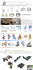### chemical equation - Waterford Schools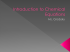### CrystalMaker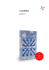### Page 13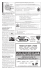### Inorganic Materials Database for Exploring the Nature of Material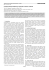### our world at natures coast landings rv resort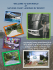### The Tower of Voices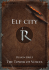### We Get Atom-ized, and It`s Outrageous### HSC Chemistry, Maharashtra Board, Paper - 1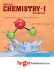### Dalton`s Atoms and ! Gay-Lussac`s Laws! of Combination"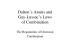### Class 4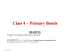### Mini Lingual Spur Educator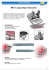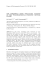### Hydrogen Storage on Calcium-Coated Toroidal Carbon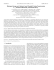### The Orgone Generator Bible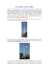### Layout 5 - The Room at Coulter`s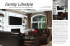### Teacher`s Resource Guide - The Molecularium Project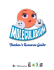### PDF of article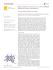### Effect of Solvent on Crystal Habit and Dissolution Behavior of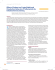### Ultracold Atoms in Line-World: Bose